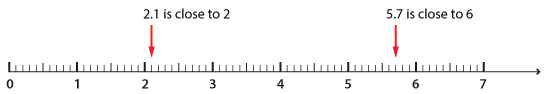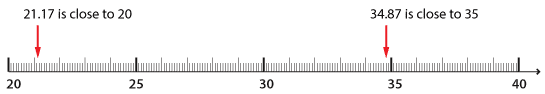#### Checking the reasonableness of answers

Before we even begin to calculate with decimal numbers, we should use strategies to estimate an answer. Estimation helps us understand if our answer is reasonable or 'in the ball park'. Being able to make 'calculated guesses' or estimations is a vital skill in everyday life, as well as a way to check for the reasonableness of the results of calculations at school.

With a simple calculation such as 5.7 + 2.1, we round off each number to the nearest whole number and then add to give an estimate. 5.7 is rounded to 6 and 2.1 is rounded to 2. So our estimate is 6 + 2 = 8.

The use of a number line helps to make this more evident:Detailed description

If the numbers being added involve hundredths, the number line can clearly demonstrate how to round to the nearest whole number:Detailed description

34.87 + 21.17 =

34.87 is rounded to 35 and 21.17 is rounded to 21.

35 + 21 is equal to 56.

When we do the calculation, we can see that the estimate is close to the answer:

 3 4 . 8 7 + 2 $$1_1$$ . $$1_1$$ 7 5 6 . 0 4